### Brevet Pajak Online UnsoedBrevet Pajak Online Unsoed  dibuka untuk 25 peserta setiap kelas. Informasi lebih lanjut hubungi  Reva (0858-8579-8331) atau  Ida (0821-3378-9932) - WA/call/SMS. Pendaftaran dibuka sepanjang waktu. DAFTAR ONLINE SEKARANG! Brevet Pajak Unsoed Reguler akan kembali dilaksanakan setelah situasi dan kondisi memungkinkan. Kunjungi dan ikuti Brevet Pajak Unsoed Purwokerto di Facebook  dan Instagram untuk mendapatkan informasi terbaru mengenai pendaftaran, acara, dan aktivitas kami Pengertian brevet pajak Unsoed Brevet Konsultan Pajak Fakultas Ekonomi dan Bisnis Universitas Jenderal Soedirman, selanjutnya disebut brevet pajak Unsoed , adalah program kursus/pelatihan pajak yang diselenggarakan oleh Laboratorium Pengembangan Akuntansi Unsoed dengan mengacu kepada SK Rektor Unsoed No. KEPT.276/UN23/PP.08.00/2017, sebagai salah satu unit layanan yang berada di lingkungan FEB Unsoed. Hingga saat ini (awal 2018), brevet pajak Unsoed telah diselenggarakan sebanyak 64 angkatan dengan ribuan alu

### What is depreciation in accounting: definition and meaning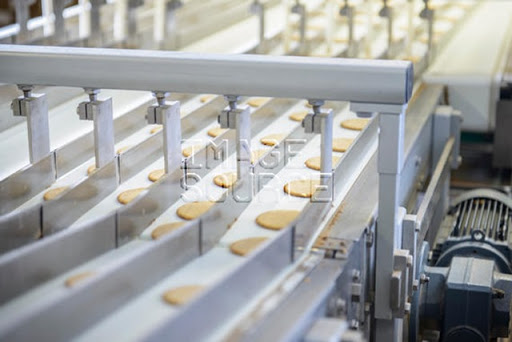Depreciation is the systematic allocation of the depreciable cost of an asset over its useful life (depreciation definition per IAS 16). Journal entry for depreciation is usually made up of a debit to depreciation expense and a credit to accumulated depreciation.

## Depreciation definition

What is depreciation? Depreciation in accounting has a specific meaning. The term depreciation refers to “the systematic allocation of the depreciable amount of an asset over its useful life”. Let me explain each concept in the depreciation definition to make it clear.

IAS 16 defines the term depreciable amount as “the cost of an asset, or other amount substituted for cost, less its residual value.” To avoid unnecessary confusion, it is not needed for now to think of what is the other amount that can be substituted for cost. If the residual value is considered to be zero, then depreciable amount, also often called depreciable cost, is simply the asset’s cost.

What is the meaning of useful life? IAS 16 defines the useful life of assets as either the period over which the assets are expected to be available for use; or the number of production or similar units expected to be obtained from the assets. Therefore, useful life is defined in terms of time or activity level. For example, a building is expected to be available for use for the periods of 20 years, while another asset, a piece of machinary is expected to be available to produce 20,000 units of finished goods.

Why the cost of an asset should be allocated over its useful life? Not all assets should be depreciated. Depreciation in accounting is required only for some long-lived tangible assets, such as buildings, cars, computers and machinary. Those assets are expected to generate services for many years.

If a company records the entire cost of such assets as expenses on the statement of profit or loss for the period in which they are acquired, it would make the company reports a considerable loss, even if the investment itself is a sound decision.

Fortunately, accounting adopts an expense recognition principle called the matching principle. The matching principle means that efforts (expenses) should be matched with results (revenues). Because long-lived assets provide benefits over more than one accounting period, it is meaningful to allocate the cost over their useful life. That is the reason for depreciation accounting. In other words, depreciation in accounting is a process that results in the cost of assets spread out over the periods in which the assets are being used.

At the end of the accounting period, a journal entry for depreciation is needed for allocating the cost of long-lived tangible assets that has been used in the current period (depreciation expense) on the statement of profit or loss, and reporting the unused cost (an asset) on the statement of financial position (balance sheet). The adjusting journal entry for depreciation is usually made up of a debit to depreciation expense and a credit to accumulated depreciation.

What is accumulated depreciation? Accumulated depreciation is the total cumulative depreciation that has been charged to an asset as it is being used by a company. In other words, the balance in the accumulated depreciation account represents the total cost that has been allocated over the life of the asset.

So, what kind of account is accumulated depreciation? Is accumulated depreciation an asset? The accumulated depreciation account is called a contra asset account. After the adjusting journal entry for depreciation is posted to the related accounts, the ending balance of accumulated depreciation appears on the statement of financial position (balance sheet). It is offset against the related asset. The result of such offsetting is called the carrying amount or the book value.

The fact that the term book value refers to the difference between the cost of the asset and the accumulated depreciation, depreciation is not a matter of valuation at all. That is why IAS 16 prefer to use the term carrying amount. In this post, I use both terms interchangeably. So, when in one occasion I say carrying value, it is exactly the same as when I say book value in another ocasion.

IAS 16 defines book value or carrying amount as the amount at which an asset is recognized (on the statement of financial position) after deducting any accumulated depreciation and accumulated impairment losses. The definition makes it clear that book value is merely the amount of unamortized cost of an asset. It has nothing to do with the value of an asset.

## Simple depreciation example

To shed light on the meaning of depreciation, let’s me give you a simple depreciation example. Assume that on January 1, 2016, Frontier Advertising Company (FAC) purchased some pieces of equipment to be used in the office. The cost of the equipment was \$5,000 in total. FAC would make a journal entry to record the purchase of equipment.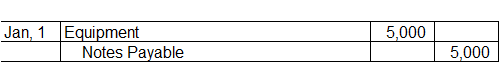If it was the first equipment the company had ever purchased and there was no more debit or credit to the equipment account during the year, the balance would be \$5,000 debit.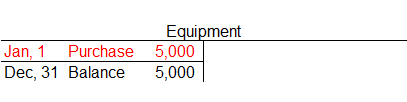Assume that FAC used straight line depreciation on the equipment, with estimated useful life of 10 years and \$200 residual value. How to calculate depreciation on the equipment? The depreciation expense would be \$480 a year [(\$5,000 cost - \$200 residual value) ÷ 10 years estimated useful life]. The adjusting entry for depreciation on the equipment at the end of the accounting period would be::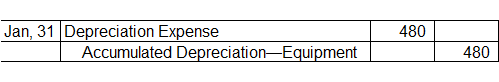When the debit to depreciation expense was posted to the depreciation expense account in general ledger, the depreciation expense account would have a debit balance of \$480.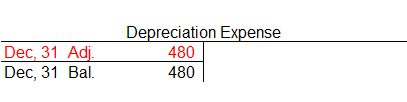After FAC prepared the statement of profit or loss, the ending balance of the depreciation expense, a temporary account, would be closed/transferred to an equity account (e.g. the owner’s capital or retained earnings account).

As discussed earlier in this post, the accumulated depreciation account is a real account, disclosed as a contra asset account in the statement of financial position (balance sheet). The ending balance of the accumulated depreciation account is carried forward to the next accounting period.

The balance of FAC’s accumulated depreciation account would be \$480 at the end of the accounting period.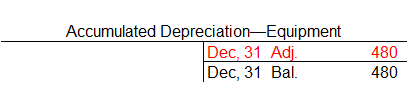As you see in this depreciation example, depreciation in accounting is an allocation concept, not a valuation concept. Depreciation merely allocates an asset’s cost to the periods in which it is used, does not attempt to report the actual change in the value of the asset.

The carrying amount of FAC’s equipment is \$4,520 (\$5,000 cost - \$480 accumulated depreciation) as at December 31, 2016. Again, the carrying amount does not represent the value of the equipment at the end of the period. In the US, it is commonly called book value.

The presentation of the cost of equipment, accumulated depreciation, and carrying amount would be: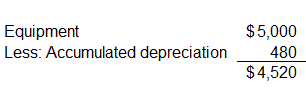At the end of the next accounting period, the same adjusting journal entry for depreciation is repeated. On December 31, 2017, FAC would make a debit of \$480 to the depreciation expense account and a credit of \$480 to the accumulated depreciation account. Because the accumulated depreciation is a real account, the balance would be \$960 (\$480 + \$480), hence the book value of the equipment as at February 28 is \$3,560 (\$4,520 - \$960).

The adjusting journal entry for depreciation of an asset will come to the end when the depreciable amount (also commonly called depreciation basis) has been fully recognized on the statements of profit or loss during the useful life of the asset.

Now you can also see that depreciation is a noncash expense. The credit in the journal entry for depreciation is to the accumulated depreciation account, not to the cash account. Depreciation also does not provide resources for the replacement of assets. When a company prepares statement of cash flows, the depreciation expense will be added back to profit or loss in the computation of net cash flows from operating activities.

## Is depreciation always an expense?

Our depreciation example so far shows that the adjusting journal entry for depreciation is a debit to depreciation expense and a credit to accumulated depreciation. On the statement of profit or loss, the depreciation expense is reported under the operating expenses’ section and the accumulated depreciation is a contra asset account disclosed to arive at the carrying amount of the asset as recognized on the statement of financial position (balance sheet).

Is it always the case? Is depreciation always reported using the label “depreciation expense” and classified as operating expenses?

The quick answer is “No!”. Why? For a manufacturing company, depreciation on the long-lived tangible assets used in the production process is part of overhead costs. Overhead costs consist of costs that are indirectly related to the production of finished goods. Other examples of overhead costs include indirect materials, indirect labor, insurance, taxes, and maintenance on factory facilities.

Using the numbers from depreciation example above, if the equipment is part of the factory facilities, the adjusting entry for depreciation would be: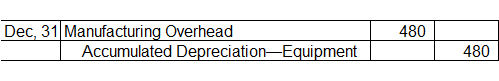The manufacturing overhead is a temporary account to accumulate various indirect costs in the production process. The adjusting entries in a manufacturing company includes the assignment of those costs to work in process inventory as illustrated below: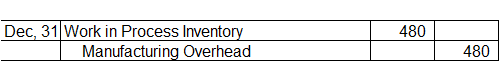The adjusting journal entry is intended to assign the manufacturing overhead related to the depreciation on equipment during the current period to the work in process account. Of course, the manufacturing overhead account would also include other indirect costs incurred during the period, not only the depreciation amount.

Work in process inventory is a real account to accumulate the costs of units that are partially completed at the end of the accounting period. When the units are completed, the costs are transferred to the finished goods inventory account. In other words, there are three common types of inventories in a manufacturing company, i.e. raw materials, work in process, and finished good inventories.

Being the result of the allocation of an asset’s cost, depreciation amount is then reallocated to other types of assets (e.g. to work in process inventory and finally to finished goods inventory). When the finished goods are sold to the customers, depreciation would then be part of the cost of goods sold, another type of expenses, reported on the statement of profit or loss.

## Depreciation methods: how to calculate depreciation?

Our depreciation example in this post assumes that the depreciation expense incurred is \$480 a year. What does it mean? Where does the depreciation amount come from? In fact, our discussion so far does not consider a very important point in depreciation accounting: the depreciation methods. We have not discussed what is straight line depreciation as implied in the depreciation example.

Depreciation accounting assumes that the useful life of an asset is limited as either a function of time or a function of activity level. As time passes, technological changes can make an asset become obsolete. Normal deterioration also limits the use of an asset. Two methods based on this assumption are called straight line depreciation and accelerated depreciation. For some assets, level of activity limits their physical usage. The units of production method of depreciation is based on this assumption.

Upcoming tutorials will discuss in more detailed those depreciation methods.

## Conclusions

The main purpose of adjusting entry for depreciation is to recognize the use of assets during the current period. The adjusting entry for depreciation is usually made up of a debit to depreciation expense account and a credit to accumulated depreciation account (or to related asset account directly).

However, this is not always the case. For example, if the reporting entity (the company) is a manufacturer, or is constructing its own facilities, depreciation charged to the long-lived assets contributing to the production or construction processes can be part of the overhead costs. In this case, depreciation is a component of product costs.

The amount of depreciation charged depends on which depreciation method is chosen by a company. Depreciation methods can be categorized into two broad classes based on their assumption. If depreciation is a function of time (for example the depreciation of buildings, furniture and computers), straight line or accelerated depreciation methods is considered to be a rational choice. If depreciation is a function of activity level, the units of production method of depreciation can be more appropriate.

Last updated: April 1, 2017